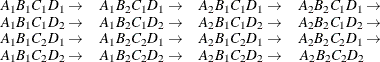General Effects

An example that combines all the effects is X1*X2*A*B*C(D E). The continuous list comes first, followed by the crossed list, followed by the nested list in parentheses. You should be aware of the sequencing of parameters when you use statements that depend on the ordering of parameters. Such statements include CONTRAST and ESTIMATE statements, which are used in a number of procedures to estimate and test functions of the parameters.

Effects might be renamed by the procedure to correspond to ordering rules. For example, B*A(E D) might be renamed A*B(D E) to satisfy the following:

• Classification variables that occur outside parentheses (crossed effects) are sorted in the order in which they appear in the CLASS statement.

• Variables within parentheses (nested effects) are sorted in the order in which they appear in the CLASS statement.

The sequencing of the parameters generated by an effect can be described by which variables have their levels indexed faster:

• Variables in the crossed list index faster than variables in the nested list.

• Within a crossed or nested list, variables to the right index faster than variables to the left.

For example, suppose a model includes four effects—A, B, C, and D—each having two levels, 1 and 2. If the CLASS statement is

class A B C D;

then the order of the parameters for the effect B*A(C D), which is renamed A*B(C D), isNote that first the crossed effects B and A are sorted in the order in which they appear in the CLASS statement so that A precedes B in the parameter list. Then, for each combination of the nested effects in turn, combinations of A and B appear. The B effect changes fastest because it is rightmost in the cross list. Then A changes next fastest, and D changes next fastest. The C effect changes most slowly because it is leftmost in the nested list.